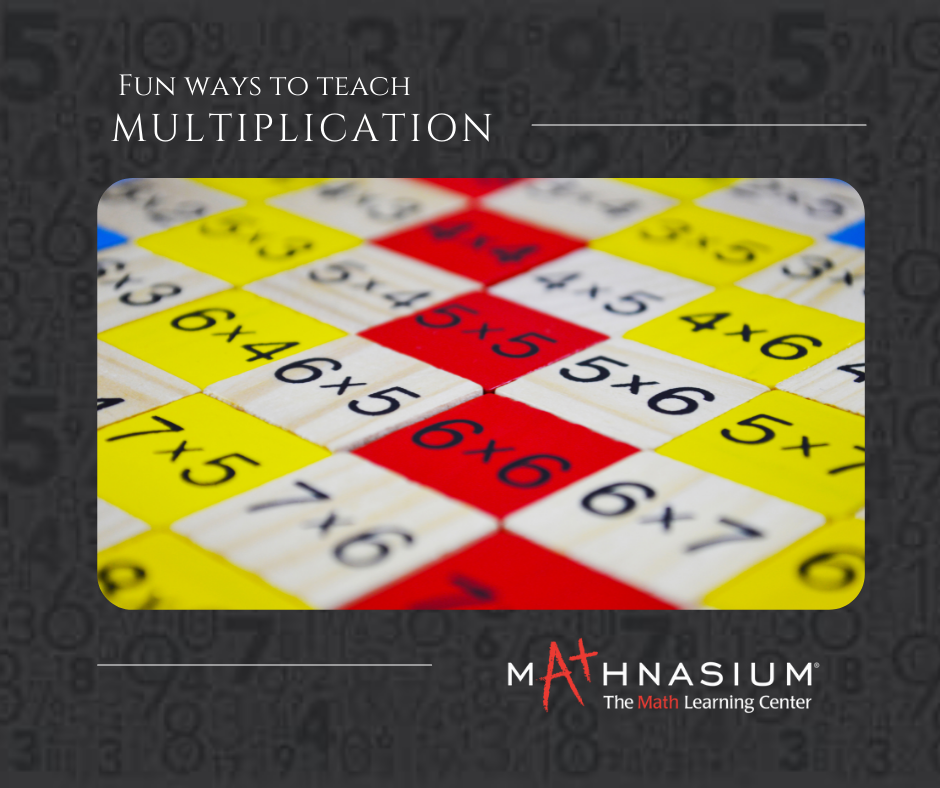877-601-6284
Get Started by Finding a Local Center

# FUN WAYS TO TEACH MULTIPLICATION

Jan 2, 2023 | Snoqualmie ValleyMany students see multiplication as a complete set of problems and fail to understand the basic multiplication facts. Many students focus on memorizing answers instead of understanding the concepts that brought them to that answer. If those numbers come easily, they will tackle more complicated and advanced problems with ease. For better understanding, they should understand how multiplication operates. So, review the idea that multiplication is repeated addition, or in other words, several groups of equal amounts are added.

The traditional drill route of memorizing multiplication tables is not fun. So here, we are sharing some fun, hands-on ways that make multiplication fun and easy to understand.

Start Concrete, Move On to Abstract When first introducing multiplication, provide plenty of manipulatives to gain a strong visual grasp of the concept. Then slowly introduce real-world examples or word problems.

For example: Ammie has 4 cartons of eggs. Each carton has 3 eggs. How many eggs are there? Once kids are familiar with real-world problems, make the connection to multiplication. Using the example above, you can begin explaining like this “I have 5 groups of eggs. Each group has 3 eggs. That’s 5 x 3. I have 3 eggs five times. It’s multiplication!”

Use Manipulatives Manipulatives are a wonderful tool to teach kids how to organize multiplication. They can use anything from beads, blocks, paper clips, or whatever you find around the house to understand math concepts. “Build a rectangle with 3 columns of 4 squares” demo cards.

For example, in the above problem, a student must organize the squares on the right into an array of 3 columns of 4 squares each.

Skip Counting Another way to think of multiplication is with skip counting.

For example, you can say, “We are skip counting by 3s while doing jumping jacks.” The kids get out of their seats and do a jumping jack for “3, 6, 9, 12” and so on staying in a sequence of 3s. Being up and moving helps to keep the blood circulating and increases memory. It also makes skip counting fun!

Practice Multiplication Rules A good way to help students understand multiplication is to have them understand some rules. These consist of:: Any number multiplied by one remains the same. Any number multiplied by two is just that number plus itself. Any number multiplied by 10 gets a zero on the end. Any number up to nine multiplied by 11 is the same digit repeated twice. If you reverse the numbers in a multiplication problem you will get the same result. For eg 3x5 and 5x3 both equal 15. This is called the commutative property of multiplication. Once students understand the commutative property, they will begin to spot it in problems, helping them to evaluate it more easily. They’ll also have an easier time memorizing their tables because learning one fact means you also learn its inverse.

Work with words It’s best to introduce word problems alongside fact fluency practice, so students understand how multiplication translates to real scenarios. The shift to words can be tricky, so ease students in by visualizing the problem, to begin with. Provide illustrations of the quantifiable aspects of the problem, or help students draw them themselves.

Learning multiplication facts can be a daunting task, but there are better ways to learn besides grueling memorization sessions. With these strategies and tools in mind, you can see how multiplication doesn’t have to be a scary or daunting subject for your students. When broken down into digestible constituents, you will avoid the frustrations that frequently accompany the practice of learning multiplication. Use these ideas to reinforce a well-structured math curriculum, and watch your students’ learning outcomes improve.

We hope this blog gives you some fun ideas for your kid's multiplication learning!

##### Locations near
Could not find Center, try again